SYLLABUS  Previous: 2.2 Explicit 3 levels  Up: 2 FINITE DIFFERENCES  Next: 2.4 Leap-frog, staggered grids

## 2.3 Lax-Wendroff

Slide : [ Lax-Wendroff Scheme - Code - Run || VIDEO login]

Rather than counting on your intuition for the right combination of terms, is it possible to formulate a systematic recipe for solving an equation in Eulerian coordinates and with any chosen level of accuracy? Yes, using the so-called Lax-Wendroff approach, which can easily be generalized for non-linear and vector equations.

1. Discretize the function on a regular grid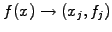,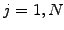,
2. Expand the differential operators in time using a Taylor series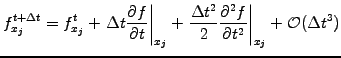(2.3.0#eq.6)

3. Substitute the time derivatives from the master equation; for the case of advection (1.3.1#eq.1), this yields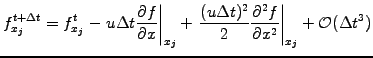(2.3.0#eq.7)

4. Use centered differences for spatial operators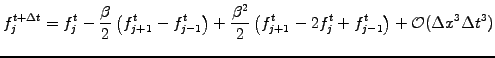(2.3.0#eq.8)

The example here yields a second order Lax-Wendroff scheme for advection that is explicit, centered and stable provided that the CFL number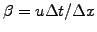remains below unity:
      for (int j=1; j<n; j++) {
fp[j]=f[j] -0.5*beta *(f[j+1]-f[j-1])
+0.5*beta*beta*(f[j+1]-2.*f[j]+f[j-1]); }
fp=f -0.5*beta *(f[ 1 ]-f[ n ])
+0.5*beta*beta*(f[ 1 ]-2.*f+f[ n ]);
fp[n]=f[n] -0.5*beta *(f[ 0 ]-f[n-1])
+0.5*beta*beta*(f[ 0 ]-2.*f[n]+f[n-1]);


The JBONE applet below illustrates how the Lax-Wendroff scheme combines the properties of the 2 and 3 levels schemes from the two previous sections.

 Numerical experiments: explicit Lax-Wendroff Examine the propagation and the damping of harmonic functions; try to assess the numerical properties directly from your observations. Test if this scheme also works for negative velocities. Explain what happens when choosing the magic time step for which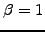; why can you not rely on this in an inhomogeneous medium?

SYLLABUS  Previous: 2.2 Explicit 3 levels  Up: 2 FINITE DIFFERENCES  Next: 2.4 Leap-frog, staggered grids